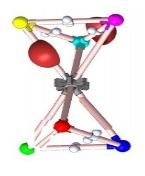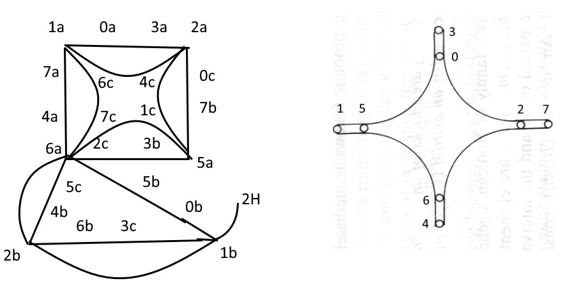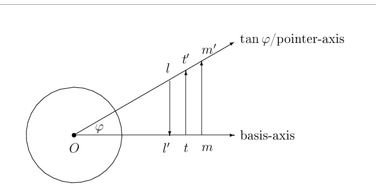## Acta Scientific Women's Health (ISSN: 2582-3205)

#### Short CommunicationVolume 3 Issue 3

Octonians and the EM-Compass

Gudrun Kalmbach HE*

Mathematic Informatics Naturewissenchaften, Technic, Germany

*Corresponding Author: Gudrun Kalmbach HE, Mathematic Informatics Naturewissenchaften, Technic, Germany.

Received: February 02, 2021; Published: February 26, 2021

Citation: Gudrun Kalmbach HE. “Octonians and the EM-Compass”. Acta Scientific Women's Health 3.3 (2021): 57-60.

## Abstract

In the first section, the EM compass is described and an application to leptons. Since the octonians are used, a Hilbert subspace lattice structure is described: why the extension from spacetime coordinates to octonians necessary.

Keywords: EM-Compass; Octonians

EM-compass

The EM-compass is the 8th roots version of the G-compass which is the 6th roots version of a dihedral compass. If octonians are generated by doubling the quaternions, the two leptonic series are listed with their EM properties as signed rotating EM (neutral) charge, magnetic momentum (momentum) and induction (oscillation) and the octonians are marked as 8th roots of unity on the EM-compass circle with the eight properties added. In comparison, for the G-compass six color charges are added to the 6th roots of unity (Figure 4). The matrix G is of order 6, the matrix i1/2 is of order 8. It has as scalar 1/√2 and the first row of the matrix B is (1 -1), the second row (1 1). For the G-compass the conjugation operator associated a color charge x = r,g,b with its dual c(x) turquoise, magenta, yellow and the Heisenerg uncertainties did arise: x, c(x) are drawn as two rays on a coordinate axis u as directions u, -u where u is the coordinate x, φ, t and u is momentum, angular momentum, energy.Figure 1: Deuteron with two nuceon tetrahedrons, red r is the x-axis, green the y-axis, blue the z-axis.

For the EM-compass the weak decay associates with e+ (positron) the neutrino ν, with e- the antineutrino c(ν), magnetic momentum μ the momentum p = mv and with the EM induction the (anti-)neutrino oscillation. The last pair is for an octonian EM-compass: For the G-compass the octonian first e0 and last e7 coordinates have been used as a (eigen)vector setting units for measures or angles θ for two rays at a common origin with this angle in between and e7 was rolled to a Kaluza-Klein circle for the complex polar exponential function exp(iφ) as circumference U(1) of the compass. In this sense, induction is a cross product vector for e0 as rotational EM momentum, area differentiated from a magnetic flow (or reversely the flow integrated from induction). The U(1) circle (e7) is replaced by an oscillation as g = exp(i(ωt+φ0)),

t time, ω = dφ/dt angular speed, φ0 a phase angle. The oscillation is interpreted as a pendulum motion for neutrinos (or antineutrinos) in figure 4. The former datas (ν,p,g) are weights of a Gleason frame GF orthogonal base triple with quaternionic coordinates, attaching Pauli matrices to the triple (ν,p,g) and adding the identity matrix. The p, pj vector has three values for neutrinos three masses mj. The oscillation g is discrete, cyclic with the B2 matrix powers as 1,i,-1,-i and back to 1. At rest, the observable neutrino is (ν,p1,g) with the smallest mass. In the first application of g, substituting φ = π/2 for (ωt+φ0) for the turn of the compass needle, 1→i a Higgs field for mass makes the triple (ν,p2,g) observable, then in sequence i→(-1) (ν,p3,g) is observable, (-1)→(-i) (ν,p2,g), (-i)→1 (ν,p1,g). The Higgs field is replacing an added rgb-graviton to three quarks in a nucleon where a similar SI-rotor is postulated.

The oscillation corresponds to it. This is not named a WI-rotor since it applies for neutral leptons, but not for the electrical charged EM leptons. For them the Hopf fiber bundle makes another spherical, 2-dimensional model as rotating sphere S2 (Figure 2). On the north pole ∞ of S2 sit the spin plus attached magnetic momentum (replacing p) vectors for the rotation axis towards the south pole 0 of S2, on a latitude circle C rotates an EM charge as pole P, replacing the g oscillation, at 0 a Higgs field sets the mass at rest for the EM lepton. In a Hopf blow up to the weak S3 sphere, points of S2 become fibers S1 (replacing U(1)), 0 is the core to which the EM torus for C can be retracted, the leaning circle for P is rotating about a central torus z-axis and spin has a 45 degree leaning angle θ towards the z-axis.Figure 2: EM lepton S2.Figure 3: Orbitals for electrons in atoms show their different Hopf locations as sphere, club, torus; at right the inverse Hopf map geometry of S2 in S3; in the middle the helicity of neutral leptons with spin s aligned with momentum p.Figure 4: G-compass at left, magnetic symmetry (middle), pendulum oscillation at right.

Hilbert space octonians

The eight dimensions of octonians have a complex meaning for a Hilbert space presentation H4 dimension as complex 4-dimensional space C4. The lattice L of (closed) subspaces consists as in the real case R4 of Boolean blocks 24 where all subspaces and their corresponding projection operators are commuting. Noncommuting means that L is the set theoretical union of its blocks. A block is drawn as an interval with four atoms marked for base vectors or lines in H4. For H = R these are local coordinates spacetime (x,y,z,t), for H = C octonian coordinates ej with j = 1,2,3,4 for these coordinates and complex with (z1 = (x,e5), z2 = (y,e7),z3 = (z,e0),z4 =(t,e6)). This doubling of coordinates for the blocks in C4 has a meaning for keeping the lattice structure of H4 where the projections make the splitting of H4 in two orthogonal subspaces U + U, for wεC as the complex presentation w = x + iy. For a 4-cycle (figure 5 at left) in L there must exist a central flash (Figure 5 at right).Figure 5: A sublattice diagram with a 4-cycle and a 3-cycle, flash with octonian coordinate indices; the lower triangle at left shows that in L this requires an additional block, containing its vertices.

In the flash two adjacent blocks have two atoms in common. The coupling is 15 for the Heisenberg uncertainty position-momentum (x,e5) and 46 time energy (t,e6). The Heisenberg uncertainty angle angular momentum is not presented. It is replaced by (2,7) and (0,3). The last case gives a new EM-compass uncertainty with maximal bound speed of light c for universes matter speeds [1-11].

In case an uncertainty for (2,7) is wanted, the mass rescalings for matter systems can be quoted.

As measuring GF it is (2,5,7). Mass can be renormed through Minkowski metrical computations through relative speed between two coordinate systems (using a sin φ =v/c rescaling), additive rescaled by measuring inner frequencies for speeds/energies in the system through mc2 = hf or observed in mass defects. The coordinate 7 is then a ψ function for it and is observable through a probability distribution ψc(ψ). As a wave package for a system, the Minkowski rescaling gives a common (optical computed) group speed with which it moves in the environment. The former neutrino oscillation is another form of observing their different masses. A Higgs field uses different approaches for attributing mass to a system and for setting barycenters of combined systems where a newly computed mass is set. The weak decay allows that an intermediate barycenter of a weak boson is annihilated and two generated systems from it get two (or no) different barycenters. For two systems, when a gravitational interaction is generated for them, a common barycenter is set and used by Higgs for attributing mass to the new system. The Schwarzschild radius Rs of a system is used when it belongs to a huge system Q (as sun) and an orbiting system P (as planet). It sets for rescaling time as differential in the tangent space a dt rescaling angle sin β = Rs/r as dt‘ = dt∙cos β.

In both cases, Schwarzschild and Minkowski the area between a space and time coordinate is preserved, dr‘dt‘ = dr∙dt, l‘t‘ = l∙t. For dr the rescaling is then dr‘ = dr/(cos β). Both rescalings use orthogonal projections beween two rays in an angle β or φ (Figure 6). For β the mathematical inversion of a mass system at the Schwarzschild radius of a black hole inverts its radius r in r‘r = Rs2 when absorbed. The angle φ speed v is inverted for dark energy systems in v‘v = c2. At the borders, the electromagnetic waves have speed v = c and Higgs bosons in fields may use r = Rs. The normed Schwarzschild radius matrix is G of order 6 from the G-compass, having first row (1 -1) and second row (1 0). B of order 8 for the EM-compass has the second row replaced by (1 1).Figure 6: Minkowski watch for rescaling of length, time, mass as orthogonal, spiralic projection.

The Minkowski scaled matrix M of order 2 has the columns of G interchanged, as Moebius transformation it is (-v + c)/c, G is (r – Rs)/r and for B the scaling factor is 1/√2 as proportion between the first and second cosmic speed of a matter system. B2 is the real Pauli matrix σ2 with first row (0 1) and second row (-1 0). In case a two ray angle is wanted for B, it is the i1/2 45 degree angle, for G it is replaced by 60 degree and for m 180 degree. The 8th, 6th or square roots belong to characteristic polynomials zn -1, n = 8,6,2. Difference equations replace differential equations and allow 8,6, or 2 solutions. For M the speed v can be signed to +v or -v for Lorentz transformations.

All matrices use as rows different bases in a plane E. B2 is for presenting complex numbers z = x + iy in matrix form z = x∙id + y∙σ2 with first row (x y) and second row (-y x). The quaternionic extension Q of C uses for σ1, σ3 other bases of E. Euler angles generate the quaternions noncommutative Pauli matrices.

As a research project is posed: look at the operator or matrix transformations between the different 2 x 2-matrices in use. Included in the study can be the 3 x 3-GellMann matrices of SU(3), extended from Pauli matrices. In complex form, the above matrix for complex numbers as 2 x 2-matrix is in quaternions the matrix with complex numbers z1 = z +ict, z2 = x +iy, in the first row (z1 z2), in the second row (-c(z2) c(z2)). In SU(3) matrices z3 = (m,f) = (e5,e6) i added as cross product z3 = z1xz2 and the three 4-dimensional spaces are in matrix form written, having a last row and column with 0 coordinates added or inserting them in the second or first position. Their first rows are then (z1 z2 0), (z1 0 z3), (0 z2 z3). The octonian cross product z4 = z1xz2xz3 is missing. The extension from SU(2) to SU(3) allows another multiplication table than the octonians and other measuring triples GF.

## Bibliography

1. G Kalmbach HE. "MINT-WIGRIS". MINT Verlag, Bad Woerishofen (2017).
2. G Kalmbach HE. “(Chef-Hrsg), MINT (Mathematik, Informatik, Naturwissenschaften, Technik)”. MINT Verlag, Bad Woerishofen, volume 1-65 (1997-2020).
3. Internet video under YouTube: Moebius Transformations Revealed (2014).
4. G Kalmbach and U Eberspaecher. “MINT-Wigris Tool Bag”. Bad Woerishofen (2019-2021).
5. G Kalmbach. “MINT-Wigris Postulates”. In: researchgate.net under MINT-Wigris Project (2020).
6. E Schmutzer. “Projektive einheitliche Feldtheorie”. Harry Deutsch, Frankfurt (2004).
7. K Stierstadt. “Physik der Materie”. VCH, Weinheim (1989).
8. G Kalmbach HE. MINT-Wigris project. In the internet under: researchgate.net.
9. Kalmbach G. “Orthomodular Lattices”. London New York: Academic Press (1983): 390.
10. T Poston and I Stewart. “Catastrophe theory and its applications”. Pitman, London (1978).
11. G Kalmbach HE. “A Heisenberg c-compass”. Journal of Applied Material Science and Engineering Research 2 (2020): 63-67.

Copyright: © 2021 Gudrun Kalmbach HE. This is an open-access article distributed under the terms of the Creative Commons Attribution License, which permits unrestricted use, distribution, and reproduction in any medium, provided the original author and source are credited.

## News and Events

• Certification for Review
Acta Scientific certifies the Editors/reviewers for their review done towards the assigned articles of the respective journals.
• Submission Timeline for Upcoming Issue
The last date for submission of articles for regular Issues is March 20, 2023.
• Publication Certificate
Authors will be issued a "Publication Certificate" as a mark of appreciation for publishing their work.
• Best Article of the Issue
The Editors will elect one Best Article after each issue release. The authors of this article will be provided with a certificate of “Best Article of the Issue”.
• Welcoming Article Submission
Acta Scientific delightfully welcomes active researchers for submission of articles towards the upcoming issue of respective journals.